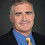## Wednesday, May 31, 2006

### Dispersing Ramsey Graphs

Perhaps the very first example one sees of the probabilistic method: Show there exists a graph on n vertices with no clique or independent set of size k = 2log n. Simply pick the graph at random. For any set S of k vertices the probability that the graph restricted to S will be a clique or independent set is at most p=2-(k choose 2)/2. The probability that any subset S is a clique or independent is at most p times (n choose k) which is less than one for k = 2log n. So there must be some graph with no clique or independent set of size k.

Actually constructing such a Ramsey graph is another story. You can create the graph in quasipolynomial (2poly log n) time using standard derandomization techniques. In 1981, Frankl and Wilson had a polynomial-time construction of a graph that had no clique or independent sets of size 2(log n log log n)1/2. That bound stood until the recent STOC paper by Barak, Rao, Shaltiel and Wigderson creating a graph with no clique or independent set of size 2(log n)o(1).

Barak et. al. were not trying to create Ramsey graphs, rather to create randomness dispersers for two independent weakly random sources. As a bonus they improved the Frankl-Wilson bound giving yet another example where proving one kind of theorem in complexity gives an exciting result in an apparently unrelated area.

1.Dispersers and Ramsey graphs are essentially the same object. This is hardly an example of the kind you are looking for (a work in one area giving a result in a different area).

2.An example of the kind one is looking for can perhaps be provided by Vaughan Jones' fabled creation of his knot polynomial - he was working on vertex operator algebras and whence he realised that that work implied a new knot invariant. The connection was "sweet" all the more since it got him the Fields :)

3.But the techiques used are very different from those used previously, aren't they? Perhaps that's the point.

4.wait, this isn't quite right.

You're talking about construction of Ramsey graphs on n vertices with no monochromatic clique or independent set of size k, and give bounds on that.

But the paper is talking about bipartite Ramsey graphs on n x n vertices, which are a different thing. Here we have no monochromatic sets A,B each of size k on each side of the bipartite graph such that there are no edges between A and B. It's a different thing.

5.The authors point out that one can use a "standard transformation" to convert such a bipartite Ramsey graph to an ordinary Ramsey graph with the same parameters.

6.The "standard transformation" is this one: given a potential edge (a,b) of the Ramsey graph with a \geq b, include (a,b) in the graph iff (a,b) is included in the bipartite Ramsey graph.

Then it is easy to check that the new graph has no clique or independent set of size K if the old one avoided bipartite cliques and bip. independent sets of size K/2.

7.Isn't p=2*2^(-k choose 2) instead of p=2^(-k choose 2)/2?